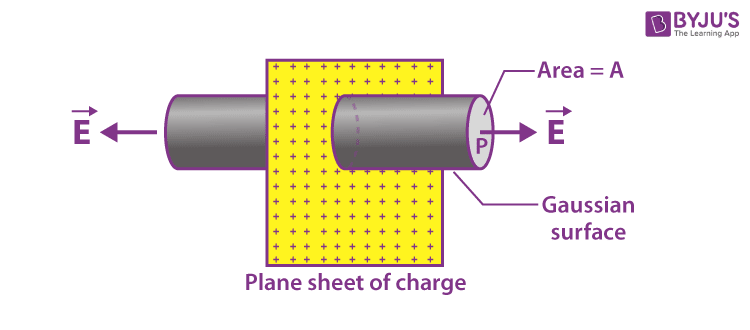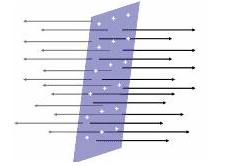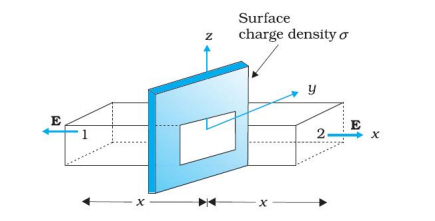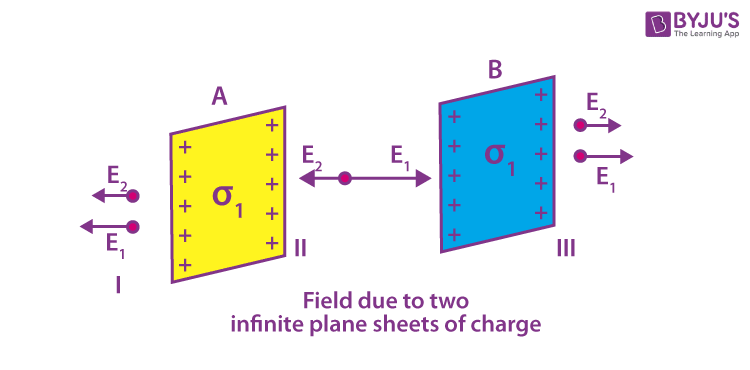# Electric Field Intensity Due To A Thin Uniformly Charged Infinite Plane Sheet

As we know, the electric force per unit charge describes the electric field. The electric field determines the direction of the field. We know that for a positive charge, the electric field is directed radially outward, and for a negative point charge, the electric field is directed inwards. Electric fields originate from electric charges or from time-varying magnetic fields. Electric fields are one of the key factors for the electromagnetic force and are one of the four fundamental forces of nature.

In this article, let us learn about the electric field intensity due to a thin, uniformly charged infinite plane sheet.The uniform distribution of the surface charge on an infinite plane sheet is represented as

$$\begin{array}{l}\sigma\end{array}$$
. In the above figure, the x-axis is normal to the given plane. The electric field does not depend on y and z coordinates, and its direction at each point shall be parallel to the x-axis.

The Gaussian surface considered in the above case is a rectangular parallelepiped having a cross-sectional area A..

The unit vector 1 is placed in -x direction normal to the surface 1 and the next unit vector is placed in the +x direction, normal to the surface 2. Therefore, electric flux over these edges

$$\begin{array}{l}\vec{E}.\hat{n} ds\end{array}$$
are equal and add up. The total flux through the Gaussian surface = 2 EA.

It is noticed that only the faces 1 and 2 will contribute to the flux (the unit vector

$$\begin{array}{l}\hat{n}\end{array}$$
and
$$\begin{array}{l}\vec{E}\end{array}$$
are parallel to each other). The electric field lines are perpendicular to the other faces and therefore, do not contribute to the total flux.

In the closed surface, the net charge enclosed is given by

$$\begin{array}{l}q = \sigma * A\end{array}$$

Hence,

$$\begin{array}{l}2EA = \frac{q}{\epsilon _{0}} = \frac{\sigma A}{\epsilon _{0}}\end{array}$$

or

$$\begin{array}{l}E = \frac{\sigma }{2\epsilon _{0}}\end{array}$$

Vectorially,

$$\begin{array}{l}E = \frac{\sigma }{2\epsilon _{0}}\hat{n}\end{array}$$

Where,

$$\begin{array}{l}\hat{n}\end{array}$$
= a unit vector normal to the plane and going away from it

The nature of charge distribution decides the direction of the electric field vector.

If,

$$\begin{array}{l}\sigma\end{array}$$
> 0, it conveys that E is directed outwards.

If,

$$\begin{array}{l}\sigma\end{array}$$
< 0, it conveys that E is directed inwards.

It is clear from the above expression that E is independent of the distance of the point from the plane charged sheet.

## Electric field Intensity Due to Infinite Plane Parallel Sheets

Consider two plane parallel sheets of charge A and B. Let

$$\begin{array}{l}\sigma1\end{array}$$

and

$$\begin{array}{l}\sigma2\end{array}$$
be uniform surface charges on A and B.Electric field due to sheet A is

$$\begin{array}{l}E_{1} = \frac{\sigma _{1}}{2\epsilon _{0}}\end{array}$$

Electric field due to sheet B is

$$\begin{array}{l}E_{2} = \frac{\sigma _{2}}{2\epsilon _{0}}\end{array}$$

=

$$\begin{array}{l}\frac{\sigma _{1}}{2\epsilon _{0}} – \frac{\sigma _{2}}{2\epsilon _{0}} = 0\end{array}$$

Note: There exists no electric field in the region between two sheets which are parallel to each other.

Stay tuned with BYJU’S for more such interesting derivations in physics, chemistry and maths in an engaging way with video explanations.

## Frequently Asked Questions on Electric Field Intensity

### 1. What is an electric field?

An electric field is defined as the electric force per unit charge and is represented by the alphabet E.

### 2. What is the formula to find the electric field intensity due to a thin uniformly charged infinite plane sheet?

$$\begin{array}{l}E = \frac{\sigma }{2\epsilon _{0}}\hat{n}\end{array}$$

### 3. How is the uniform distribution of the surface charge on an infinite plane sheet represented as?

$$\begin{array}{l}\sigma\end{array}$$

2 EA.

E = F/q.

## Electrical Force And Its Characteristics